Thermodynamics and Propulsion
Subsections

# 16.3 Steady-State One-Dimensional Conduction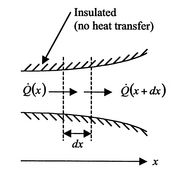For one-dimensional heat conduction (temperature depending on one variable only), we can devise a basic description of the process. The first law in control volume form (steady flow energy equation) with no shaft work and no mass flow reduces to the statement thatfor all surfaces(no heat transfer on top or bottom of Figure 16.3). From Equation (16.6), the heat transfer rate in at the left (at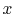) is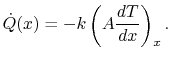(16..9)

The heat transfer rate on the right is(16..10)

Using the conditions on the overall heat flow and the expressions in (16.9) and (16.10)(16..11)

Taking the limit asapproaches zero we obtain(16..12)

or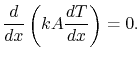(16..13)

Ifis constant (i.e. if the properties of the bar are independent of temperature), this reduces to(16..14)

or (using the chain rule)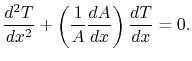(16..15)

Equation (16.14) or (16.15) describes the temperature field for quasi-one-dimensional steady state (no time dependence) heat transfer. We now apply this to an example.

## 16.3.1 Example: Heat transfer through a plane slabFor this configuration (Figure 16.4), the area is not a function of, i.e.. Equation (16.15) thus becomes(16..16)

Equation (16.16) can be integrated immediately to yield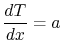(16..17)

and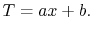(16..18)

Equation (16.18) is an expression for the temperature field where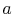and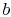are constants of integration. For a second order equation, such as (16.16), we need two boundary conditions to determineand. One such set of boundary conditions can be the specification of the temperatures at both sides of the slab as shown in Figure 16.4, say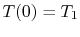;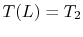.

The conditionimplies that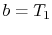. The condition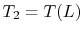implies that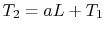, or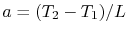. With these expressions forandthe temperature distribution can be written as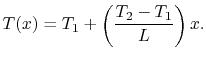(16..19)

This linear variation in temperature is shown in Figure 16.5 for a situation in which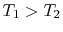.The heat flux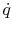is also of interest. This is given by(16..20)

Muddy Points

How specific do we need to be about when the one-dimensional assumption is valid? Is it enough to say that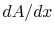is small? (MP 16.2)

Why is the thermal conductivity of light gases such as helium (monoatomic) or hydrogen (diatomic) much higher than heavier gases such as argon (monoatomic) or nitrogen (diatomic)? (MP 16.3)

UnifiedTP